IF IT'S EDUCATION, IT'S ITS
Pathways To Learning... Since 2005
Hong Kong Registered School 566985

# C - C to cyclinder - Mathematics Dictionary

A   B   C   D   E   F   G   H   I   J   K   L   M   N   O   P   Q   R   S   T   U   V   W   X   Y   Z: A symbol known as the Blackboard-bold C, commonly used to represent the set of complex numbers.∞ : A Blackboard-bold C with an infinity sign suffixed, commonly used to reger to the set of extended complex numbers, that is, the set of complex numbers together with a single point of infinity.

calculus: A branch of mathematics, the study of (infinitely many) infinitesimal quantities (which may be changing). It was classically made sense through the idea of limits and functions, thus they form an important part of the study historically.

calculus of variations: The study of extremising functionals analogous to calculus and the extremising of functions. As the set of derivatives and integrals can be thought of as forming a vector space, in addition to being analogous to, the study is naturally considered as overlapping with calculus to a certain extent.

cal: Calorie - a metric unit of energy. The equivalent SI unit of energy is joule. 1 cal (lower case c) is the energy required to raise 1 gram of water by 1 °C at roughly 4.2 joules, while 1 Cal (capital C) is the energy required to raise 1 gram of water by 1 °C 4.2 kilojoules.

cancellation: Any method of calculation the result of which, when compared to the original form, attributes a number of components of the original form to mitigate the effects of the rest (of those components), so that the result remains the same through their omission. A common example invloves the numerator and denominator of a fraction, and another invloves the omission of one logarithm symbol from each side of the equation (when the each side consists of the logarithm only), even though the former is a result of the equivalence of fractions and the latter the injective nature of the logarithmic function. Failure of understanding these can result in the misuse of such methods, and is commonplace amongst students.

canonical: Describes a representation of an object (e.g. an expression, a transformation) in a way that is preferred, perhaps unique or considered natural due to certain properties that it exhibits, even though there may be other equivalent representations.

cantilever: A beam or such similar structures which is anchored at only one end such that it resists rotation under load.

cap: An informal class="d-title" name for symbol ∩, used to denote intersection of sets that is easier to say than the polysyllabic "intersection".

capture-recapture sampling: A method for estimating total population (usually of animals) through 2 periods of capture (observation), assuming that the total number has reamined constant and that the probability of capture of any animal on any one visit is constant and equal. In implementing this method, a researcher captures a number of animals and mark them (or make sure that they can be identified in the future in some way) and releases them, they come back to capture a number of animals and count the proportion of animals that were captured both times. By assuming that the proportion of animals recaptured in an unbiased estimate of the proportion of animals marked in the first capture, combine this with the number of animals marked on the first visit, we can easily calculate the estimated number of the total population. In practice, it is difficult to ensure that the animal population remains constant (or roughly the same) while ensuring that the chance of recapture is truly equal amongst the population since the former requires the visits to be relatively close to each other so that the population does not change significantly and yet the latter condition requires the visits to be sufficiently space apart so that the locations of the animals are truly randomised.

cardinal number: A number used to represent the size of a set. The abstract concept of size in this sense is called cardinality. See ordinal number.

cardinal points: North, East, South and West, the four directions equally spaced apart on the surface of the Earth. Since the idea orignated from when the Earth was generally assumed to be flat, we need to carefully define the idea of these "directions" using the idea of geodesics, since 2 lines in the same "direction", in this sense, are not necessarily parallel.

cardioid: A curve class="d-title" named for being roughly heart-shaped, though it has a cusp, it has no point (of sudden change in direction) at the opposite point on the loop. It is the locus traced out by a fixed point on a circle, as the circle rolls around another circle of equal radius and fixed centre.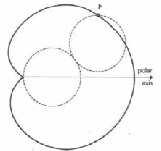Cartesian coordinate system: The rectilinear coordinate system used for any (finite and natural) number of dimensions. The number of axes used is commonly seen as a basic way of "defining" the number of dimensions.

These axes must all be mutually perpendicular and the components (the coordinates) of a point are the shortest distances of a point to the hyperplane consisting the axes (except the axis related to the component in question). Thus, the x-coordinate of a point is the shortest distance from the point to any point on the line (plane) consisting of the y-axis (both y and z-axes) in 2D (3D).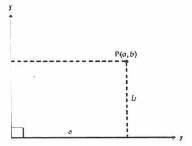While rectilinear coordinate system is a more descriptive class="d-title" name of its function, it is more commonly called Cartesian, class="d-title" named after Cartesius, the Latin class="d-title" name of René Descartes.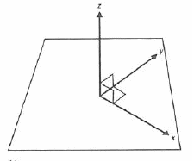Cartesian product: The Cartesian product of two sets is the set of all ordered pairs such that the first element of the ordered pair is an element of the first set in the product and similarly, the second element of the ordered pair is an element of the second set in the product.

casting out nines: A method of verification of integer arithmetics by checking that the answer matches the equivalent calculations under modulo arithmetic. The modulus of 9 is chosen simply because of our decimal systems (base 10). The difference of 1 between the modulus (9) and the base (10) allows for an easy conversion of a number to modulo 9. (By adding the constituent digits of a number represented in base 10.)

categorical data: Data that is used (or can only be used) as labels rather than quantities, as such no arithmetic structure exist and certain concepts (such as mean or median) are undefined.

categorical variable: A random variable with values which are categories. (e.g. nationality, gender etc.)

catenary: A plane curve which, if any sections of it are used to represent a string of uniform mass, has the lowest gravitational potential energy for the given length and fixed points. (Assuming constant gravity.) Thus, it is approximately the shape of a string hanging under gravity. (It is only an approximation since gravitational strength varies at different height.)

The class="d-title" name is derived from thr latin word for chains, as it is observed to be the shape of hanging chains historically.

catenoid: A surface formed by the rotation of a catenary.

Cauchy convergence condition: 1. For sequences - a necessary and sufficient condition for the convergence of a sequence: an infinite sequqnece is convergent, if and only if, for any positive number ε, there is always a number N(ε) (N is a function of ε), such that the difference between any two terms after the Nth term is less than ε.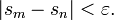where m and n are integers greater than N.

2. For series - a necessary and sufficient condition for the convergence of a series: An infinite series Σan is convergent, if and only if, for any positive number ε, there is an integer N(ε) (N is a function of ε), such thatfor all integers n > N and p is a positive integer.

Cauchy distribution: A symmetric continuous probability distribution with infinite support.

Cauchy ratio test: Also known simply as the ratio test. A method of deciding the convergence of a series through the general ratio of one term of the series to the next.

Cauchy-Riemann equations: A system of partial differential equations logically equivalent to the condition of holomorphicity of a differentiable function in complex analysis. For z = x + iy and f(z) = u + iv: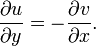Cauchy's integral theorem: A theorem that states that, a complex function f(z) which is holomorphic and is integrated along two paths with the same endpoints are the same. Thus the integral of a complex function f(z) holomorphic along the closed curve γ is always zero.Cauchy's residue theorem: Mostly known as the residue theorem, it is the generalisation of Cauchy's integral Theorem and Cauchy's Integral formula.

cause variable: Also known as an independent variable, an explanatory variable, a predictor or predictor variable or regressor (see regression).

caustic: The envelope of rays refracted or reflected by a geometric object.

Cavalieri’s principle: Two geometrical figures whose cross sections are the same as each other, at the same distance away from some reference line/lines (plane/planes) have the same area (volume). As an example, this explains why triangles whose bases have the same length, and have the same height, have also the same area, regardless of its shape.

Cayley table: The rectangular array listing all possible results of a binary operation on finitely many elements (usually of a group).

c.d.f.: Cumulative distribution function of a probability distribution.

ceiling function: A function on real numbers whose value is always rounded up, if the argument is not already an integer. The function leaves integers unchanged.

celestial mechanics: The study of motions of celestial bodies such as stars, planets and comets etc.

cell: Categories of data divided into rectangular arrays through more than one variable. It is essentially a conventional use for the analogue of groups in 1 dimensions.

Celsuis: Represented by the symbol °C. It is based on dividing the difference between the freezing point and boiling point of water into 100 equal "degrees:.

censored observations: In statistics, observations which are made incomplete systematically due to the nature of the procedure (possible) for observation or the objects under study.

census: A survey of the entire population.

centesimal measure:A metricangular measure dividing a right angle into 100 centesimal degrees.

centi-: An SI prefix for one one hundredth.

central angle: An angle between two radii (of a circle or sphere).

central conic: A conic section with a centre - a hyperbola or an ellipse (which includes the case of a circle). i.e. all the conic sections except the parabola.

central difference: The absolute or directed difference between values whose argument are a fixed equal interval away from the nominated argument.

central force: A force whose direction is always toawards the centre, and whose magnitude is a function of the distance between the point of application and the centre.central limit theorem: The theorem which states that the mean of a large number of independent random variables tends to a normal distribution as long as it satisfies certain conditions. It essentially explains the prevalence of the normal distribution.

central polyhedral angle: The angle between a number of planes all containing the centre of the sphere.

central tendency: A common measure in summary statistics bsed around the loose idea that we can assign one location to represent the locations of a number of objects considered as one. Thus, there is not just one but rather a number of slightly different concepts which fits the description of central tendency. It is what is commonly referred to by the similarly loose idea of an average.

centre (centre of symmetry): A point about which a geometric figure is in some way self-similar. It can either be a centre of rotational symmetry, or the intersections of multiple lines/planes of reflective symmetry, or even the intersections of medians in a triangle.

centre of a group: The set of elements which are commutative with every elements of the group. Note that there must be at least one element in the centre, the identity. Whereas the centre can be as large as the group, such a group is called an Abelian group.

centre of buoyancy: The centre of gravity of the body of water that an object displaces.

centre of curvature: Given a curve, the centre of curvature of a point on this curve is the centre of a circle which "locally" (for a neighbourhood of that point) describes the curvature of the curve.

centre of gravity: A point through gavity can be considered to be acting, instead of individually on the point masses or acting on the body as a whole. It is a different concept from the centre of mass, although due to the small differences in gravitational strength in most context, they can be considered close approximates of each other.

centre of mass: The point (not necessarily within the object) which is the weighted average of the point masses of a body (or the set of infinitely many point masses through integration), which can be used to calculate linear motion of a rigid body as if all of the object's mass are at that point (the centre of mass) only. (Considering the object as a particle.) It is also known as the barycentre.

centre of rotation: The invariant point in a rotation.

centripetal component: A component of an object's acceleration cooresponding to a centripetal force.

centripetal force: A force perpendicular to the velocity of an object which causes the object to travel on a curved (not straight) path.

centroid: The geometric centre of a plane figure.

cevian: Any line segment joining a vertex of a triangle to a point on the infinite line containing the oppositeside.

c.g.s. units: A system of units based on centimetres, grams and seconds. (Instead of metres, kilograms and hours for the SI units.) It has been largely superseded by the use of SI units nowadays.

chain: A set of elements and a total order.

chain complex: A sequence of (integer)-indexed Abelian groups together with a set of homomorphisms between adjacent groups satisfying certain conditions.

chain rule: A rule that finds the derivative of a composite function, given that the derivatives of the two components of the composite function can be found.

change of variable: A method for integrating a function with respect to a variable other than the one original given in the integral.

characteristic equation: Another class="d-title" name for the characteristic polynomial.

characteristic function: Another class="d-title" name for the indicator function. A function which returns a value of 1 if the argument is a member of the set specified and 0 otherwise.

characteristic polynomial: det(A-kI) = 0 , a polynomial derived from a swuare matrix A whose solutions are the eigenvalues of the transformation represented by the matrix.

Chebyshev: Russian mathematician known for his work in probability and statistics. Also transcribed as Tchebyshev.

Chinese postman problem: The problem of finding the least weighted circuit in a connected graph. If an Eulerian cycle exists than such a cycle is the solution to the problem, otherwise, it is necessary to repeat at least one edge and the problem can be more complicated.

chi-squared distribution: A distribution on the value of the sum of squares of n standard normally distributed random variables.

chi-squared test: A test on the goodness of fit of an observation to the theoretical value/assumed distribution through the use of the chi-squared distribution to test its likelihood of deviation due to natural variations.

chord: A line segment joining one point of the circumference of a circle to another. If the chord also goes through the centre than it is a diameter.

circle: The locus of all points which is equidistant from a fixed point on a plane.

circle of convergence: The set of points which is of a fixed distance from a fixed point (thus forming a circle) on an argand diagram which are the the set of arguments which converges in an infinite series.

circle of curvature: A circle which describes correctly the curvature of a curve for the neighbourhood of a point on the curve.

circuit: A closed path on a graph.

circular: Of or related to a circle.

circular data: The class of cyclic directional data in statistics.

circular functions: Another class="d-title" name for the trigonometric functions. Trigonometric functions are based on parametrization of a circle just as hyperbolic functions are based on parametrization of hyperbolae. This usage highlights the analogue between the two.

circular helix: A helix with constant radius (from an axis).

circular measure: Also known as angular measure.

circular motion: The motion of an object whose path forms a circle.

circumcentre: The centre of a circle which goes through all vertices of a polygon.

circumcircle: A circle whose circumference contains all points of the polygon.

circumference: The length of the closed curve of a circle.

circumscribed: The act of enclosing a geometric figure with a (minimal) circle or sphere.

cis: A commonly defined function in the study of complex numbers. cis(θ)=cos(θ)+isin(θ) Incidentally, cis(θ)=exp(iθ). As such, cis is seldom used apart from a particular stage of learning in school as most favour the use of the simpler exp(iθ).

Clairaut's equation: A family of differential equations of the form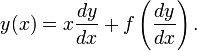which can be solved by differentiating the whole equation.

class: A collection of objects, not necessary a set. The distinction generally is that certain operations on sets are not allowed so as to allow us to refer to a (usually large) collection of objects without causing contradictions such as Russell's paradox.

class frequency: The number of occurrence in a class.

classical mechanics: A loosely defined term generally considered to be the study of motion of objects before the drastically different quantum mechanics. Thus classical mechanics include Newtonian, Lagrangian, Hamiltonian and arguably, relativistic mechanics.

class intervals: The intervals in which data fall into a particular class.

closed curve: A curve with no endpoints, generally used to refer to non-self-intersecting curves.

closed interval: An interval of real numbers containing its endpoints. More generally, it is a bounded set of points with a total order which is identical to its closure.

closed region: A set of points containing all limit points and is connected.

CM: An abbreviation for the centre of mass of an object.

coaxial: The property of having the same axis.

codomain: The codomain of a function is a superset of the image of the function. It is sometimes known as the range of the function. The codomain serves as a constraint as to what values a function can take, thus there can be elements of the codomain which is not the value of the function but not vice versa.

coefficient: The quantity with which we multiply the variable in question.

coefficient matrix: A matrix formed from a system of linear equations using the coefficients of the equations only (omitting the variables). Also known as an augmented matrix

coefficient of concordance: A measure of concordance. With m orderings and n objects, let an object i be given a rank of ri,j by the ordering j, then object i's total rank can be calculated by:while the mean of these total ranks is given byWe can caluclate the sum of squared deviations (S) bythe we can calculate the coefficient of concordance bywhich has a value between zero and 1 and a high value indicates high level of agreeement.

coefficient of correlation: A class="d-title" name for several related methods which measure the relationship between two sets of data.

coefficient of friction: A dimensionless quantity, the ratio of the friction force to the normal reaction as determined by a number of other factors. (Such the the type of surfaces in contact.)

coefficient of kurtosis: A measure of how much the data concentrates around the mean. Informally, it measured the "pointiness"/"peakedness" of the distribution curve.

coefficient of determination:A measure of how much of the variation in the data can be accounted for by the statistical model, for the purpose of inferring the likely level of determination of outcomes.

coefficient of restitution: A dimensionless quantity, the ratio of the speed of separation to the speed of approach of 2 objects as determined by other factors. (e.g. the material the objects consist of and their arrangement.)

coefficient of skewness: A measure of the asymetry of a distribution.

coefficient of variation: A measure of dispersion (standard deviation) normalised by the mean. (The absolute value of the mean is taken for a real variable to prevent the coefficient from being negative.)

cofactor: For a square matrix, augmenting the minors with a sign (positive or negative) in a "chequered" fashion forms the cofactors.

Formally, the cofactors arewhere Mij are the minors of the matrix.

cofunction identities: A set of trigonometric identites which relates trigonometric functions to their cofunctions, e.g. sin and cos, sec and csc, tan and cot.coincident: The property of 2 geometric figures to have all points in common.

colatitude: The angle between the vector and the polar axis (z-axis) in a Spherical polar coordinate system, whereas latitude is the value subtracting colatitude from 90 degrees, the smallest angle between the radius vector and the plane perpendicular to the polaw axis through the origin. (the equator)

collision: The interaction of 2 objects with each other through contact transitioned from a state of non-contact prior.

column: A vertical array of elements having all but one indices in common.

column rank: The number of linearly independent columns (considered as column vectors) in a matrix. See rank.

column vector: A matrix representation of vectors using a matrix with one column. (an nx1 matrix)

combination: The way of ways that a collection of k objects can be picked from a number of n objects. (If the objects are considered to be picked the same way at the same time so that there is no way to distinguish the ways an object is picked.)See permutation.

combinatorics: The mathematical study of counting and countable structures.

common denominator: The common multiple of the denominators of a number of fractions. A common way to add and subtract fractions is to convert each fraction into the equivalent fraction with the common denominator.

common difference: The constant which is the difference between each term and the next in an arithmetic sequence/series.

common factor: Also known as a common divisor. It is an integer which completely divides the two or more numbers in question.

common logarithm: Given a number, the common logarithm is the value such that if 10 is raised to such a value, gives exactly the number in question.

common multiple: An integer that is simultaneously a multiple of two or more numbers.

common ratio: The constant which is the ratio of one term to the next in a geometric sequence/series.

common tangent: A line which is the tangent of points on 2 distinct curves.

commutative: The property of a binary operation such that its operands can always be swapped around without affect its value.

compass: Also known as a pair of compasses, to avoid confusion with the instrument for telling directions. A pair of compasses is an instrument with rigid (but movable) arms for drawing circular arcs.

compatible matrices: Two matrices in a particular order so that they can be multiplied. In the usual convention, the number of columns in the first matrix and the number of rows in the second matrix must be the same. In more abstract terms, considering the matrices as transformations, it amounts to maintaining that the number of dimensions (rank) of the codomain of the first transformation be the same as the dimensions (rank) of the domain of the second transformation.

complement: The complement of a set is the set of elements not in the specified set. Note that this definition only makes sense with a universal set defined.

complementary angles: Two angles that sum to a right angle. In this case, each angle is called the complement of the other angle.

complementary function: Along with the particular integral, it forms the general solution of a linear differential equation. It is essentially an element in the kernel of the differential operator.

complete induction: Also known as strong induction. This method assumes that the statement is true for all values below a certain finite value in the inductive step of proving the next statement. Logically strong induction is equivalent to weak induction and is not "stronger" in this logical sense.

complete lattice: A poset where all subsets have a supremum and an infimum.

completing the square: A method for solving quadratic equations in general by writing a quadratic expression in the vertex form. Note that the quadratic formula is derived from the method of completing the square for the general quadratic expression.

complex analysis: The study of complex variabled functions.

complex conjugate: Given a complex number, the complex conjugate is the complex number whose real part is the same, while the imaginary part (being a real number) has opposite signs. The significance of complex conjugates stems from the theorem that says the complex conjugates of all roots of real polynomials are also roots themselves.

complex fraction: A fraction consisting of complex numbers. Considering the division of complex numbers as complex fractions is a standard way of calculating the division. (Through algebraic methods such as the difference of two squares.)

complex function: A function involving complex numbers. Note that, while it is true that all real variabled functions are complex functions, certain results (such as convergence) differs depending on which kind we consider the function to be.

complex number: The set of numbers which is algebraically complete with respect to finitely many additions, multiplications, exponentiation and their inverse operations. It is a superset of the real numbers.

complex plane: The collection of complex numbers represented on a plane. It is also known as an argand diagram.

component (of a vector): Individual parts of a vector. (e.g. each element in a column/row vector represent a component of the vector.)

component analysis: The study of a set of data by an isometric transformation and subsequently dividing them into orthogonal components.

composite function: The result of composing two functions together, so that the value (output) of the first becomes the argument (input) of the second. The argument of the first function is considered the argument of the composite function and it is the value of the second function that is considered the value of the composite function.

composite hypothesis: As opposed to a simple hypothesis. In hypothesis testing, a composite hypothesis is a hypothesis where the value of some parameters of the distribution is not specified to be a certain value.

composite number: A positive integer that is not a prime number. Note that 1 is conventionally considered neither prime nor composite - in that sense, a composite is a positive integer with more than 2 factors, while prime has exactly 2. The number 1, having only one factor, is thus considered neither.

composition: The act of combining 2 mathematical objects in some way. (e.g. of functions, transformations or geometric figures.)

compound distribution: The compound probability distribution is the result of a probability distribution whose parameters are distributed along other probability distributions.

compound fraction: A fraction that consists of another fractions as its numerator or denominator.

compound interest: The calculation of interest payments taking into account of previous interest payments as part of the principal.

compression: A class="d-title" name for a transformation where a figure becomes proportionaly smaller.

concave: A geometric figure where it is possible to form a line between 2 points in the figure where the line consists of points not from the figure. For a plane figure, it is equivalent to a shape having an interior angle of greater than 180 degrees.

concave function: A function whose graph is such that, for any two points of the graph, the function for arguments between the 2 points are higher than the straight line joining the 2 points. For a differentiable function, it is equivalent to a function with a monotonically decreasingly gradient.

Note that a function can be described as concave for a certain interval only.

concave polygon: A polygon, as a plane figure, which is concave.

concentric: The property of having the same centre. Usually describes circles and spheres.

conchoid: The family of plane curves (half of) whose shape resemble thre conch shells.

concordance: A measure of agreement of pairwise bijective set of orderings on a finite number of objects.

concurrent: The property of sharing a common point.

concyclic: Having the property of being on the circumference of a circle.

conditional: A mathematical sentence that describes the implication of one from another.

conditional distribution: The distribution of a random variable given that another random variable is known to be of a certain value.

conditional equation: An equation which is only true under certain contexts. Normally known simply as equations, as opposed to identities, which is true regardless of contexts.

conditional inequality: An inequality that is only true under certain conditions.

conditionally convergent series: A series that ceases to be convergent if the modulus function is applied to all of its terms before the summation.

conditional probability: The probability of an event given the (non-)occurrence of other events.

cone: A 3-dimensional geometric figure consisting of all points on line segments that joins any points in a circle (including the interior) to another point not lying on the same plane as the circle.confidence interval: An interval given as the estimate for a parameter, based on the theoretic value of the parameter given known information, while taking into account of the probability we require the actual parameter to be within the given interval.

confidence level: The probability with which a parameter should fall within the given confidence interval (Since we can never be certain due to natural variations in our observations.)

confidence region: Same as confidence interval.

configuration: A particular arrangement of mathematical objects.

confocal conics: Conic sections which share the same focus (or foci).

confounding: A certain property of an extraneous variable - the property of the so-called lurking variable.

congruence: 1. A property of similarity/sameness for 2 geometric figures. See congruent.

2. Two quantities considered the same under modulo arithmetics. See congruence modulo n.

congruence modulo n: Arithmetics where 2 quantities differing by a multiple of the chosen base n are considered the same. The two quantities are said to be congruent modulo n. An example involves the calculation of days of the week, where 5 (Friday) + 4 (2 days later) = 9 (which is congruent modulo 7 to 2 - i.e. Tuesday)

congruent: 1. One figure that would coincide with another with a combination of translation, rotation and reflection. Essentially, 2 shapes are congruent if they can be considered "the same" except for its location and orientation.

For triangles, there are certain conditions that makes it easier to decide if two such figures are the same. (Without having to consider the transformations.)

I - SSS - The length of all three sides of a triangle are the same as the lengths of the corresponding sides of another.

II - SAS - The lengths of any two pairs of sides correspond, and the angles between those sides are also the same as each other.

III - ASA/AAS - Any pair of angles on one shape correspond with the other shape, while any side of either shape is the same as the corresponding side of the other.

IV - RHS (also known as LH) - Both triangles are right-angled, their hypotenuses are of the same length and they share another side of the same length.

2. See congruence modulo n.

congruent matrices: Two matrices A and B are congruent if A = PTBP for some invertible matrix P.

conic: Also called a conic section, it is a plane curve which is the intersection of a plane with an infinite right double cone- i.e. a circle, an ellipse, a parabola and a hyperbola. (The intersections can also be a single point, a line or 2 non-parallel lines, which can respectively be considered special cases of a circle/ellipse, a parabola and a hyperbola respectively.)

There exist alternative definitions of conics involving a focus and a directrix together with a ratio of distance from the two called eccentricity.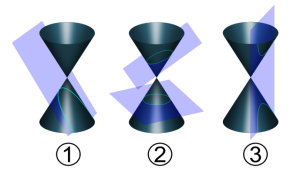conical pendulum: A weight attached through a string to a fixed point so that the trajectory of the weight is a (horizontal) circle with the string being taut (and having constant length) at all times. The circle drawn out by the weight together with the positions of the string forms a cone which explains the class="d-title" name.

conicoid: A surface generated by the rotation about an axis of one of the conics - ellipsoids, paraboloids, hypocycloids and a sphere. Also known as conoids.

conic section: Also known more simply as conics.

conjecture: An assertion that is not yet proven. In this sense, it is the same as an hypothesis.

conjugate arcs: Given an arc of a circle, the remaining section (also an arc) of the circle is the conjugate arc.

conjugate axis: The line of symmetry of a hyperbola which does not intersect the curve itself. Or alternatively, a segment of this line equal in length to the distance between the intersections between the tangent at a vertex and the two asymptotes.

conjugate complex numbers: Also known as complex conjugates.

conjugate gradient method: An iterative algorithm for the numerical solutions of certain systems of linear equations.

conjugate lines: Given a line on an argand diagram, the line formed by replace each point with its complex conjugate in known as the conjugate line. It is equivalent to a reflection of a line along the real axis of the Argand diagram.

conjugate pair theorem: A theorem which states that the complex conjugates of roots of a polynomial with real coefficients are themselves also roots of the polynomial.

conjugate points (of a conic): A pair of points on a conic which lie on the polar of the other point.

conjugate prior distribution: In Bayesian statistics, the distribution of the prior probability when the prior and the posterior distributions belong to the same family.

conjugate set: The subset of a group which are all conjugates of each other. It is also known as a conjugacy class. Note that a conjugate set is not a group since the identity must be in its own conjugate set.

conjunct: A logical operator that returns the value true if and only if both its operands are true. It essentially captures the core meaning of the connective "and" that conbines two sentences into one. Also known as a conjunction. The word conjunct has to it slight connotation towards natural languages or meta-language in the description of the (formal) logical conterpart.

conjunction: A logical operator that returns the value true if and only if both its operands are true. It essentially captures the core meaning of the connective "and" that conbines two sentences into one.

connected graph: A graph is connected if there is at least one path between any two vertices of the graph.

connected set: A set is connected if it cannot be partitioned into non-empty subsets such that each subset is disjoint from the closure of every other subset.

connective: An operator that conbines 2 sentences into a new sentence such that the truth value of the new sentence is a function of the truth values of the 2 sentences.

conoid: Also known as a conicoid.

consecutive angles: A set of angles where every member can be considered to be "next" to another angle within the set. This idea of "next" or adjacency can be sharing a side or sharing a side as well as a point depending on the context.

consecutive sides: A set of sides (edges) where every memeber can be considered to be "next" to another side within the set. This idea of "next" or adjacency can be sharing a vertex or sharing an angle depending on the context.

consequent: In the conventional way of expressing hypothetical propositions, "If A then B", the consequent is the second part of the sentence. It is the part of the sentence whose truth value is dependent on the other part. (Within the context of the statement itself.)

conservation laws: Any theorem or assertion that states that certain measurable quantities remains unchanged, and the condition under which this happens.

conservation of energy: The law that states that the amount of energy in a closed system remains unchanged. The exactness of this law, in our current understanding, depends on whether we use mass-energy as the measure or simply energy in the classical sense.

conservation of momentum: The law that states that the total momentum of a closed system of objects remains unchanged.

consistent: The property of admitting possible solutions for a system of equations.

consistent estimator: A sequence of estimators that is convergent.

constant: A quantity whose value stays constant. As opposed to a variable whose value is variable.

constant of integration: The result of apply in reverse, in integration, the fact that any constants differentiate to zero. Thus, any integrals, which can also be considered as the same integral "plus zero", integrates to an expression that always includes an arbitrary constant. Note that an arbitrary constant is not necessary in definite integration simply because whatever arbitrary value it takes, it remains a constant and the process of subtracting the value calculated with the lower bound from the value calculated with the upper bound would ensure that the effects of the arbitrary constant "cancel each other out".

constant of proportionality: The constant ratio between the change in 2 related variables said to be proportional (directly or partially) to each other.

constant term: The term in a polynomial which does not include any variables. (Note: that is not to say that the term cannot contain any unknowns.)

construction: The study of geometric figures which can be constructed using an idealised version of real world compasses and rulers (straight edge).

contact, point of: The point on a curve for which a tangent is considered to be parallel to and intersect the curve.

contingence, angle of: The angle between the directed tangents of 2 points on a plane curve.

contingency table: An array of frequencies (or relative frequencies and by extension, probabilities) recorded to study the relationship between 2 or more discrete variables.

continued fraction: A compound fraction where either the numerator or the denominator is also a continued fraction.

continued product: A product of more than 2 factors, usually analoguous to a sigma notation. Using this representation, it can be a product of infinitely many factors.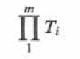continuity correction: The adjustment of the argument of a discrete distribution to form a closer approximation to a continuous argument. (Usually an adjustment of 0.5 for discrete distributions which admits integers.)

continuous distribution: A distribution where the domain of the cumulative distribution function is continuous.

continuous function A function for which a small change for every argument results in a small change in the value such that in this way, there is no limit as to how small the change in value can be.

continuous mapping A generalised analogue of a continuous function where an argument may have more than one value. (And the definition of continuous is then modified accordingly such that, a small change CAN result in a small change in the value without limits as to how small such a change may be.)

continuous random variable: A random variable whose image is continuous.

contour integral: An integral along a contour on the complex plane/argand diagram which may be parametrized.

contour plot: A type of plane graphical representation of a function with 2 arguments where sets of points of selected values form non-intersecting closed curves, known as contours.

contractible: The property of being capable of being shrunk to a point continuously.

contrapositive: Given a statement of the form "If A then B", the contrapositive of such a statement is the one which states "If not B then not A". A statement and its contrapositive are always equivalent, so that they share the same truth value.

convergence: The property of a mathematical object, considered as a sequence, is convergent.

convergent integral: A improper integral whose limit exists and is finite.

convergent iteration: An iterative process for which the sequence of each state (in order) is a convergent sequence

convergent product: A continued product whose sequence of partial products (analogues to partial sums) converges.

convergent sequence: Informally, a sequence is convergent if the terms gets arbitrarily close to a point (called the limit) so that given a proximity (that the sequence should get to), a sequence eventually gets to within such proximity without leaving it.

convergent series: A series is covergent if and only if the sequence of its partial sums is covergent.

converse: Given a statement of the form "If A then B", the converse of this statement is the one which states "If B then A". Note that the converse is not equivalent to the original statement. In fact, it is a common logical fallacy to assume that they are.

conversion period: 1. A time interval during which an investor may exchange a convertible security for another financial asset.

convex function: A function whose graph is such that, given any 2 pointst on the curve, the value of any point with argument between the 2 points lie below the straight line joining the 2 points. Note that a function can be described as convex for a certain interval only.

convex polygon: A polygon where any line segments connecting any 2 points within the polygon is also entirely within the polygon. Alternatively, it is a polygon whose angles are all 180° or less.

convex polyhedron: A 3-dimensional geometric figure where any line segments joining any 2 points are entirely within the figure.

convex set: A set of points where, given any 2 points in the set, the line segment joining the 2 points consists of points entirely from the set itself.

coordinate: One within a set of such numbers, called coordinates, which specifies the position of a point. See abscissa and ordinate.

coordinate geometry A study of geometric objects through their representation in a coordinate system.

coplanar: The property of being contained in the same plane.

coprime: The property of 2 numbers not sharing any prime factors.

corollary: A theorem which is immediate from another proven statement. That is, a theorem that requires minimally prove, or a simple observation. As such, the difference between a corollary and a theorem is not rigorously defined but rather subjective in its use.

correlation: The amount of interdependence in statistical relationship amongst two or more quantities.

cosine rule: In trigonometry,(also known as the cosine formula oe the law of cosines ) it is a statement about a general triangle that relates the lengths of its sides to the cosine of one of its angles.

countable: A set which can be put into a one-to-one correspondence with a subset of the natural numbers.

countably infinite: The property of a set being infinite and countable. For example, the set of natural numbers is countably infinite while the set of real numbers is not.

counting numbers: Also known as the natural numbers. Just as natural numbers, zero is variously included and excluded from the set of Counting/Natural numbers. Thus, it may be more precise to use the terms "positive integers" (not including zero) and "non-negative integers" (including zero) instead.

Cramer’s rule: A theorem which expresses the solutions to a system of linear equations by the determinants of matrices formed from the coefficients in the equations.

critical value: Generally speaking, a value either side of which exhibits dramatically different behaviour. Sometimes the value itself exhibits one of the 2 types of behaviour, thus making an adjacent point (if only discrete values are admitted) fitting the description also. In this case, convention usually governs which is considered to be the critical value. (As is the case in hypothesis testing, that the "first" value which rejects H0, rather than the "last" value which does not, is considered the critical value.)

cross product: More formally called the vector product - a way of multiplying 2 vectors and produces another vector perpendicular in direction to the "multiplicand" and the "multiplier". So called because we use the symbol x to denote this multiplication, as in axb. (While we use a dot, such as a.b, to denote another type of multiplication of 2 vectors - scalar multiplication/dot product.

Note that unlike the dot product, the cross product is only defined for 3-dimensional vectors. In 2-dimensions, there can be no perpendicular vectors to 2 vectors in general. (Except for the trivial case of 2 parallel vectors.) While in 4 dimensions or more, there are infinitely many vectors of a certain length perpendicular to any 2 vectors.

Thus, the vector product of 2 vectors is only defined for 2 3-dimensional vectors which are not parallel to each other.

It is called a multiplication by virtue of some of its properties being similar to multiplication in general, such as distributivity over addition and subtraction, yet, one must be careful in checking the specific properties that are the same. For example, unlike most other operations going by the class="d-title" name of multiplication (but similar to matrix multiplication), vector product is not commutative., That is, axb is never the same as bxa (except for the case when one of the vectors is the zero vector).

cube: A 3-dimensional geometric figure of 6 congruent faces (all squares) where the edges (all 12 of them) have the same length. It is the special case of a cuboid where all lengths are the same. A cube is a 3-dimensional analogue of a square just as a cuboid is a 3-dimensional analogue of a rectangle.

cube root: Given a number, the cube root of this number is another number such that the former is the cube number of the latter.

cubic polynomial: A polynomial of degree 3, that is, the highest power that the variable is raised to, amongst all terms, is 3.

cuboid: A 3-dimensional analogue of a rectangle, where all faces of a cuboid must be rectangles. A cube can be considered as a special case of a cuboid.

curve: A generally loosely defined term that describes a collection of points which form a 1-dimensional object. In specific discipline or context, the word tends to be more rigorously defined. Note that a curve can be self-intersecting, or very complicated (space-filling curve) but it must usually maintain some sense of local linearity to it to be considered a curve. (i.e. it should look like a straight line"under a microscope".)

cusp: A sudden turn in direction in a curve.

cycle: A series of events that are regularly repeated in the same order.

cycloid: The curve traced out by a fixed point on a circle (considered as a wheel) rolling along a line (considered as the ground) without slipping.

cyclinder: A right circularprism.

A   B   C   D   E   F   G   H   I   J   K   L   M   N   O   P   Q   R   S   T   U   V   W   X   Y   Z

Genius is one percent inspiration and ninety-nine percent perspiration.